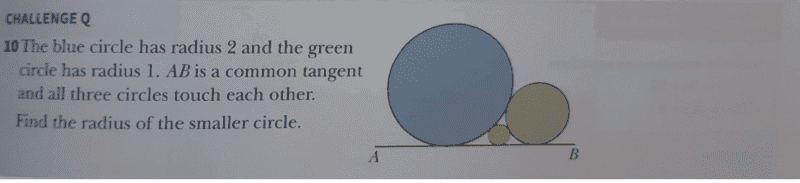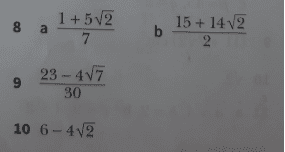# Find the radius of the smaller circle in the tangent problem

• chwala

#### chwala

Gold Member
Homework Statement
see attached
Relevant Equations
pythagoras theorem
Find the question here and the solution i.e number 10 indicated as ##6-4\sqrt{2}##,I am getting a different solution, my approach is as follows. I made use of pythagoras theorem for the three right angle triangles as follows,
Let radius of the smaller circle be equal to ##c## and distance from mid point of smaller circle to circle with radius ##1=x## then it follows that,
##x^2+(1-c)^2=(1+c)^2##
##x^2=4c##

let us also have the distance from the smaller circle to circle with radius ##2=y##, then it follows that,
##y^2+(2-c)^2=(2+c)^2##
##y^2=8c##

Let ##y^2+x^2=AB##, then it follows that, ##(AB)^2 + (2-1)^2=3^2##
##AB=2\sqrt{2}##
then it follows that, ##\sqrt{8}=2\sqrt{c}+2\sqrt{2c}##

Am i missing something here!

i got it!
##\sqrt{c}##=##\dfrac {\sqrt {8}}{2+2\sqrt{2}}##
⇒##c=0.34314575## Bingo guys! Phew i took time on this men!Last edited:
Another approach by introducing Cartesian coordinates.
Equation of circle blue
$$x^2+y^2=2^2$$
Equation of circle green
$$(x-3)^2+y^2=1^2$$
Equation of common tangent line
$$y=\frac{1}{2\sqrt{2}}(x-6)$$
Let equation of circle red be
$$x^2+y^2+2px+2qy=r$$
which is tangential to all these three figures. Thus we can have three linear equations of p, q and r, and get
$$c=\sqrt{r+p^2+q^2}$$

More simply from the figure
$$2\tan^{-1}\frac{c}{4}=tan^{-1}\frac{1}{2\sqrt{2}}$$
$$c= 4 \tan (\frac{1}{2}tan^{-1}\frac{1}{2\sqrt{2}})=4\tan (\frac{sin \theta}{1+cos \theta})$$
where ##\theta=tan^{-1}\frac{1}{2\sqrt{2}}##
$$c=4\tan \frac{1/3}{1+2\sqrt{2}/3}=4\tan (3-2\sqrt{2})\approx 0.012$$
I should appreciate if you would check whether it works or not.

Last edited:
Another approach by introducing Cartesian coordinates.
Equation of circle blue
$$x^2+y^2=2^2$$
Equation of circle green
$$(x-3)^2+y^2=1^2$$
Equation of common tangent line
$$y=\frac{1}{2\sqrt{2}}(x-6)$$
Let equation of circle red be
$$x^2+y^2+2px+2qy=r$$
which is tangential to all these three figures. Thus we can have three linear equations of p, q and r, and get
$$c=\sqrt{r+p^2+q^2}$$

More simply from the figure
$$2\tan^{-1}\frac{c}{4}=tan^{-1}\frac{1}{2\sqrt{2}}$$
$$c= 4 \tan (\frac{1}{2}tan^{-1}\frac{1}{2\sqrt{2}})=4\tan (\frac{sin \theta}{1+cos \theta})$$
where ##\theta=tan^{-1}\frac{1}{2\sqrt{2}}##
$$c=4\tan \frac{1/3}{1+2\sqrt{2}/3}=4\tan (3-2\sqrt{2})\approx 0.012$$
I should appreciate if you would check whether it works or not.
$$c=4\tan \frac{1/3}{1+2\sqrt{2}/3}=4\tan (3-2\sqrt{2})\approx 0.012$$

I am afraid this does not look correct, i overlooked your solution. I have just checked it in detail...

•anuttarasammyak

I was thinking of using linear scale factor...i do not know whether that's possible. i'll try check it out...

Let me try again. Observing angles which the triangle whose vertexes are center of circles and horizontal line on which center of orange circle lies make,
$$\alpha+\beta+\gamma=\pi$$
where
$$\sin\alpha=\frac{2-c}{2+c}$$
$$\sin\gamma=\frac{1-c}{1+c}$$
$$3^2=(2+c)^2+(1+c)^2-2 (2+c)(1+c)\cos\beta$$
This leads to ##c=6-4\sqrt{2}## which is same as OP.

Last edited:
•chwala